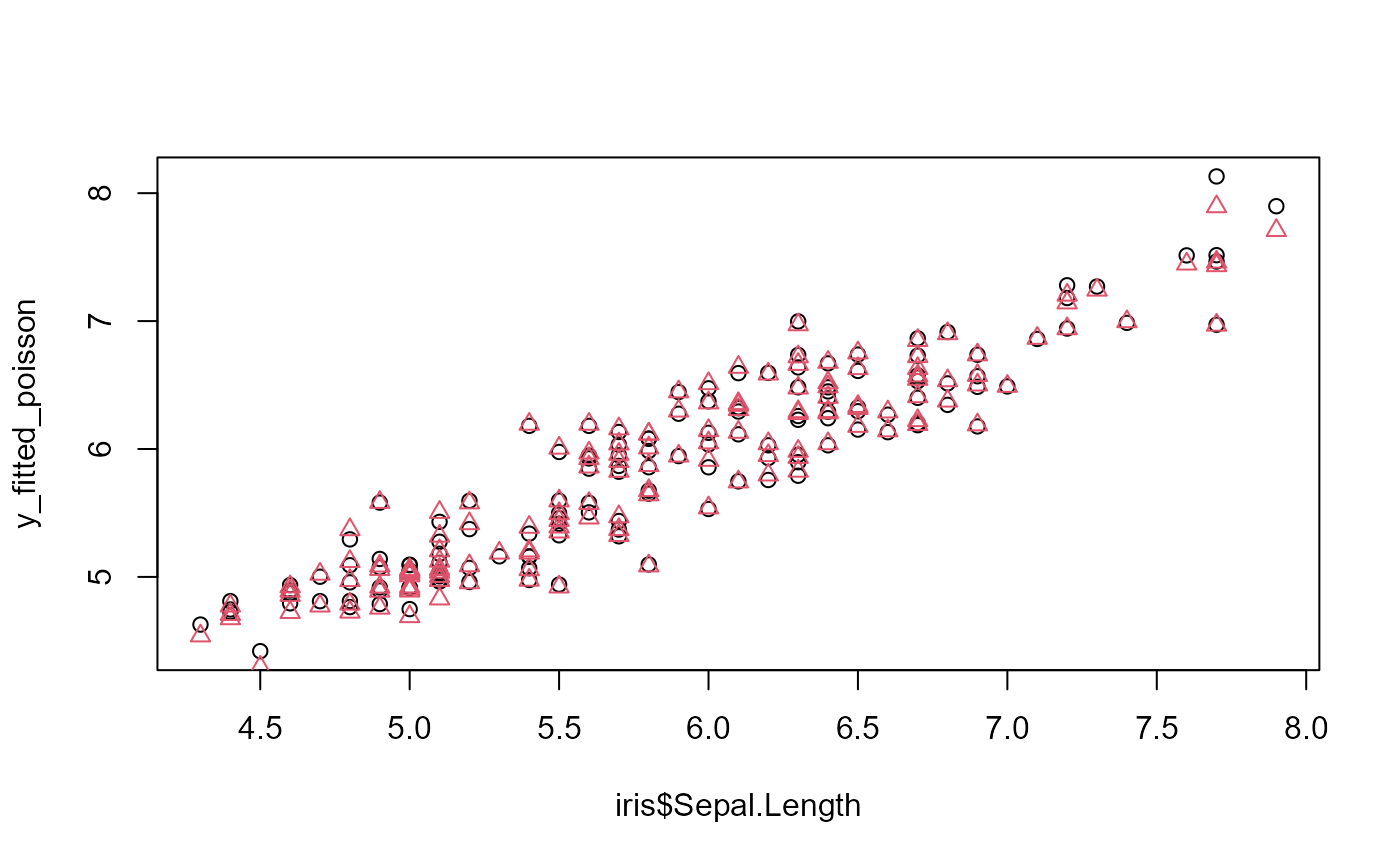This function extracts the fitted values from a model estimated with femlm, feols or feglm. The fitted values that are returned are the expected predictor.

# S3 method for fixest
fitted(object, type = c("response", "link"), na.rm = TRUE, ...)

# S3 method for fixest
fitted.values(object, type = c("response", "link"), na.rm = TRUE, ...)

Arguments

object A fixest object. Obtained using the functions femlm, feols or feglm. Character either equal to "response" (default) or "link". If type="response", then the output is at the level of the response variable, i.e. it is the expected predictor $$E(Y|X)$$. If "link", then the output is at the level of the explanatory variables, i.e. the linear predictor $$X\cdot \beta$$. Logical, default is TRUE. If FALSE the number of observation returned will be the number of observations in the original data set, otherwise it will be the number of observations used in the estimation. Not currently used.

Value

It returns a numeric vector of length the number of observations used to estimate the model.

If type = "response", the value returned is the expected predictor, i.e. the expected value of the dependent variable for the fitted model: $$E(Y|X)$$. If type = "link", the value returned is the linear predictor of the fitted model, that is $$X\cdot \beta$$ (remind that $$E(Y|X) = f(X\cdot \beta)$$).

Details

This function returns the expected predictor of a fixest fit. The likelihood functions are detailed in femlm help page.

See also the main estimation functions femlm, feols or feglm. resid.fixest, predict.fixest, summary.fixest, vcov.fixest, fixef.fixest.

Laurent Berge

Examples

# simple estimation on iris data, using "Species" fixed-effects
res_poisson = femlm(Sepal.Length ~ Sepal.Width + Petal.Length +
Petal.Width | Species, iris)

# we extract the fitted values
y_fitted_poisson = fitted(res_poisson)

# Same estimation but in OLS (Gaussian family)
res_gaussian = femlm(Sepal.Length ~ Sepal.Width + Petal.Length +
Petal.Width | Species, iris, family = "gaussian")

y_fitted_gaussian = fitted(res_gaussian)

# comparison of the fit for the two families
plot(iris$Sepal.Length, y_fitted_poisson) points(iris$Sepal.Length, y_fitted_gaussian, col = 2, pch = 2)